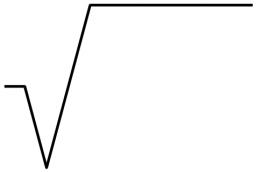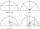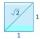# Roots

Calculate the square root of these numbers:

Correct result:

√86 =  9.27
√57 =  7.55
√6 =  2.45
√94 =  9.7
√97 =  9.85
√93 =  9.64

#### Solution:

$\sqrt{86}=9.27....9.2{7}^{2}=86$
$\sqrt{57}=7.55....7.5{5}^{2}=57$
$\sqrt{6}=2.45....2.4{5}^{2}=6$
$\sqrt{94}=9.7....9.{7}^{2}=94$
$\sqrt{97}=9.85....9.8{5}^{2}=97$
$\sqrt{93}=9.64....9.6{4}^{2}=93$We would be pleased if you find an error in the word problem, spelling mistakes, or inaccuracies and send it to us. Thank you!## Next similar math problems:

• Numeric questionIt is possible negative irrational number?
• LogsTrunk diameter is 52 cm. Is it possible to inscribe a square prism with side 36 cm?
• Transforming cuboidCuboid with dimensions 6 cm, 10, and 11 cm is converted into a cube with the same volume. What is its edge length?
• NumberIs number 5.146852 irrational?
• Expression 6Evaluate expression: -6-2(4-8)-9